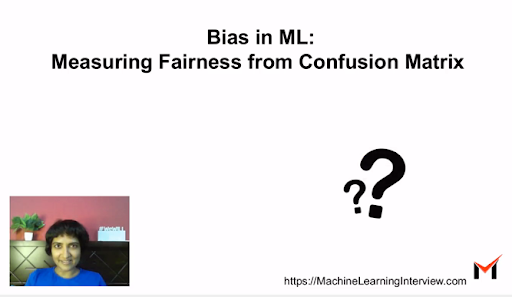# Bias in Machine Learning : How to measure Fairness based on Confusion Matrix ?

Machine Learning models often give us unexpected and biased outcomes if the underlying data is biased. Very often, our process of collecting data is incomplete or flawed leading to data often not being representative of the real world. In this article, we see why we need to measure fairness for ML models and how we can measure whether the model is fair based on metrics based on confusion matrix.

##### Why do we care about Fairness?

Lets look at a simple example. Suppose you are building a model to predict whether to approve a loan for an applicant based on features such as income level, locality, gender, education level and age.

The data might have very few women applicants who are in the high income range, who qualified for a loan earlier. However, it is unfair for the model to learn that women pose a higher risk than men from a loan perspective ! The problem here is that the gender attribute is correlated with the income attribute being low. But it is not causation, and it is wrong to learn gender as a determinant of loan risk.

##### How do we ensure ML models are fair?

To ensure ML models are fair, there are primarily three approaches:

1. PreProcessing: This involves trying to fix the data so that biases are removed from the data. For instance, remove the feature gender from the data. Or change the distribution appropriately.
2. InProcessing: Change the objective function to incorporate fairness as a objective.
3. PostProcessing: In uncertain regions, give a more favourable outcome to sensitive groups. For instance, one can have a different threshold based on gender.

However, an important aspect before we resort to one of the interventions, is to understand if the model is fair in the first place.

##### How do we measure Fairness ?

How we measure fairness depends on the task at hand. For instance, one might need to formulate a different set of metrics for a regression problem vs a classification problem vs a clustering problem.

As a popular rule of thumb, measuring fairness involves checking if the metrics we care about are similar across different groups in consideration. For instance if we care about gender bias, one could seggregage the test data into two groups by gender and measure and compare metrics across each.

##### Measuring fairness based on Confusion Matrix in classification:

Confusion matrix is a popular way of understanding how a classifier is doing, in terms of the true positives, false positives, true negatives and the false negatives. Here are some popular metrics that can be compared across various groups in question to measure fairness based on the confusion matrix:

Equal Opportunity: Is the True Positive Rate/Recall same across different groups ?
Recall that TPR indicates, of all positives, how many items we actually detected as positive. The formula for TPR is :

TPR = TP/ (TP+FN)

Equalized Odds: Is the TPR and FPR same across different groups ? In addition to TPR, this metric looks at the False Positive Rate (FPR) across groups. Recall that the FPR denotes, Out of all negatives, how many were falsely classified as positive.

FPR = FP/ (FP + TN) = False Positives / Total Number of Negatives

Accuracy: Accuracy is the fraction of correctly classified examples. It is infact the most popular classification metric. A way of measuring fairness is if the Accuracy similar across different groups.

Accuracy = (TP + TN) /  (TP + TN + FP + FN)

There could be sevaral other metrics to measure fairness, but these are a few to get started. The next section has a bunch of references if you want to digg deeper.

##### References

“Bias on the Web”, Ricardo Baeza-Yates published in Communications of ACM 2018.

Fairness in Machine Learning: A Survey: Simon Caton, Christian Haas, Oct 2020